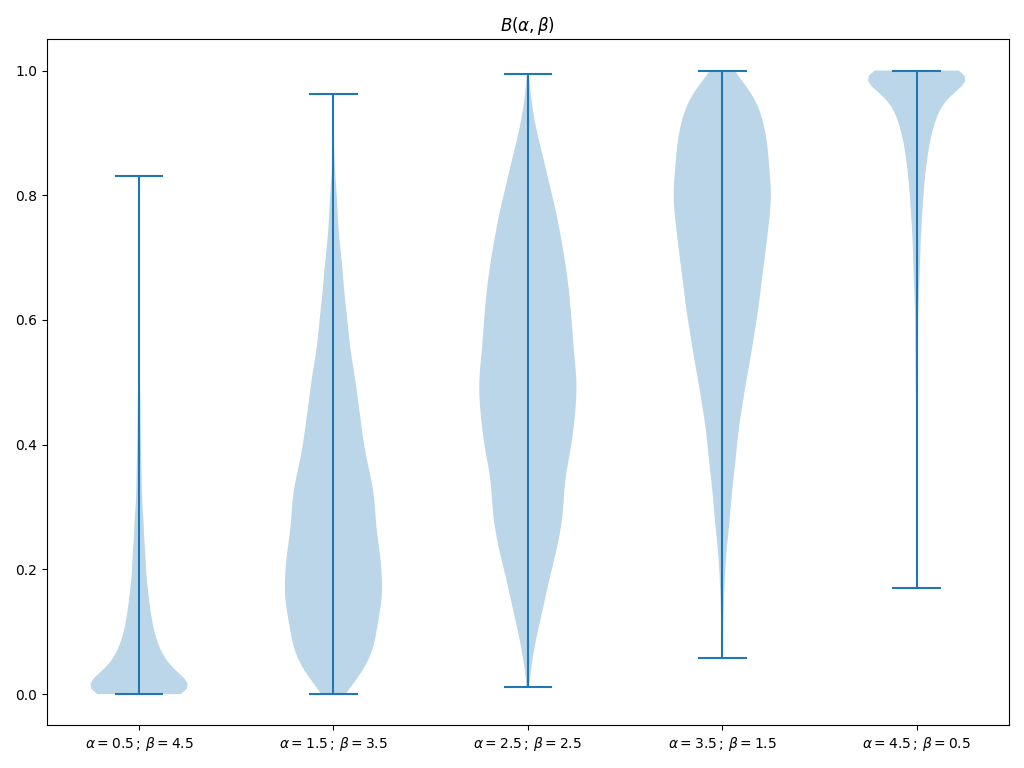# Chart Basics#

This example shows how different types of charts can be added to the scene. A more complex example, showing how to combine multiple charts as overlays in the same renderer, is given in Chart Overlays.

```import numpy as np

import pyvista as pv

rng = np.random.default_rng(1)  # Seeded random number generator for consistent data generation
```

This example shows how to create a 2D scatter plot from 100 randomly sampled datapoints. By default, the chart automatically rescales its axes such that all plotted data is visible. By right clicking on the chart you can enable zooming and panning of the chart.

```x = rng.standard_normal(100)
y = rng.standard_normal(100)
chart = pv.Chart2D()
chart.scatter(x, y, size=10, style="+")
chart.show()
```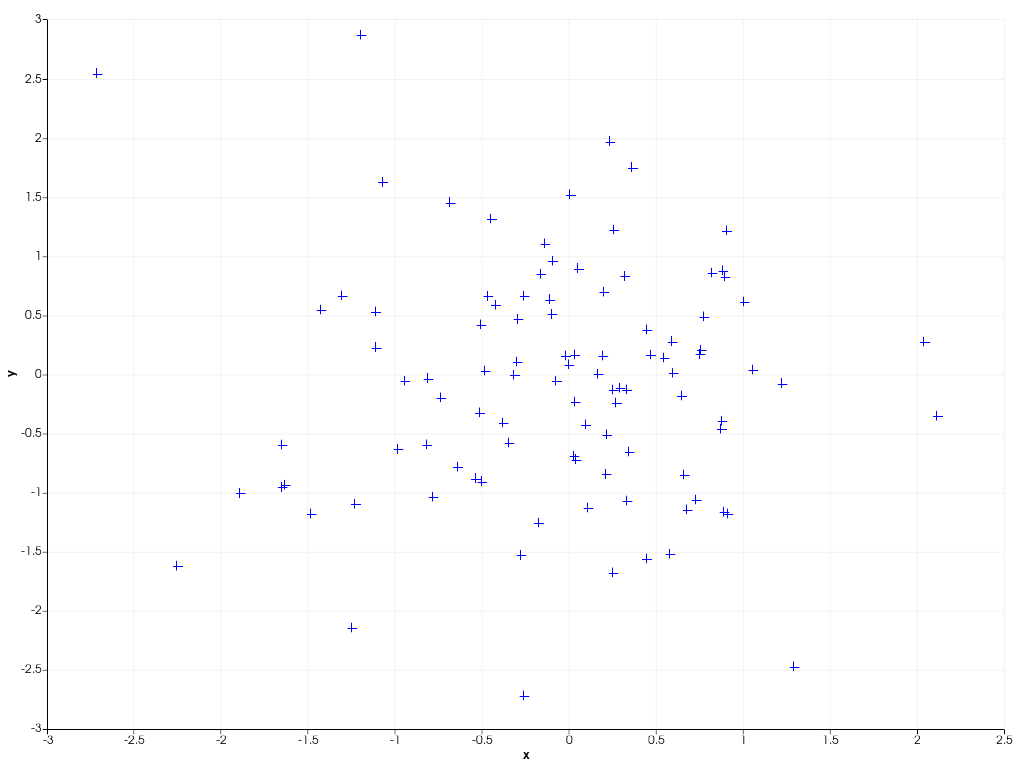To connect datapoints with lines, you can create a 2D line plot as shown in the example below. You can also dynamically ‘zoom in’ on the plotted data by specifying a custom axis range yourself.

```x = np.linspace(0, 10, 1000)
y = np.sin(x**2)
chart = pv.Chart2D()
chart.line(x, y)
chart.x_range = [5, 10]  # Focus on the second half of the curve
chart.show()
```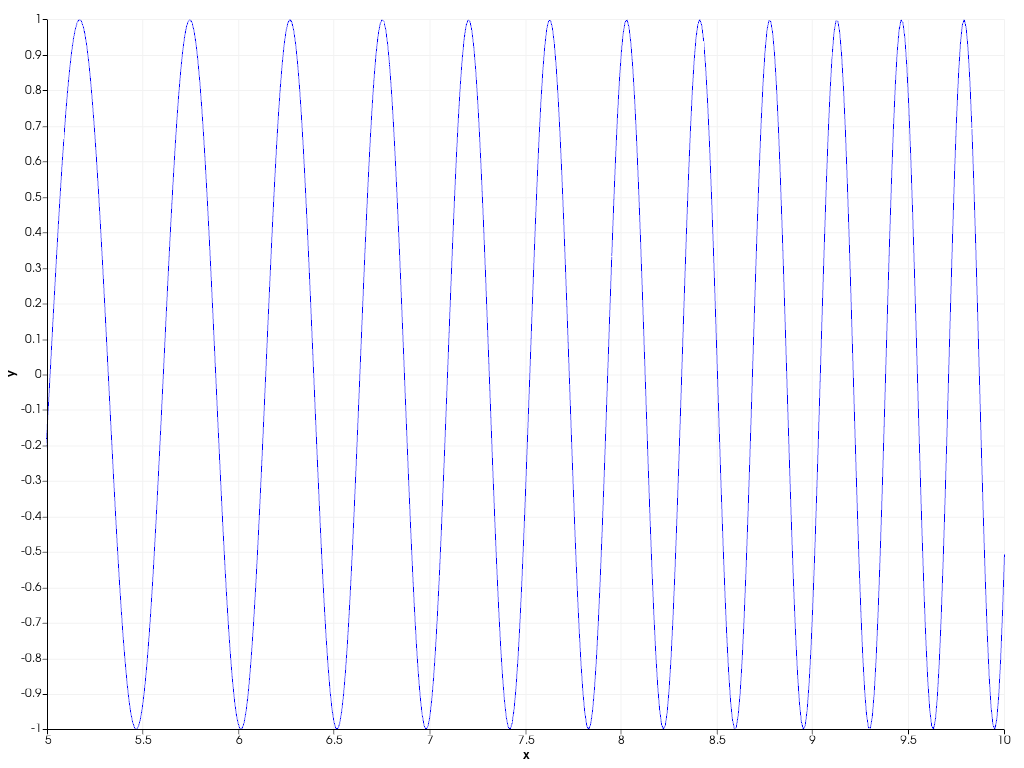You can also easily combine scatter and line plots using the general `pyvista.Chart2D.plot()` function, specifying both the line and marker style at once.

```x = np.arange(11)
y = rng.integers(-5, 6, 11)
chart = pv.Chart2D()
chart.background_color = (0.5, 0.9, 0.5)  # Use custom background color for chart
chart.plot(x, y, 'x--b')  # Marker style 'x', striped line style '--', blue color 'b'
chart.show()
```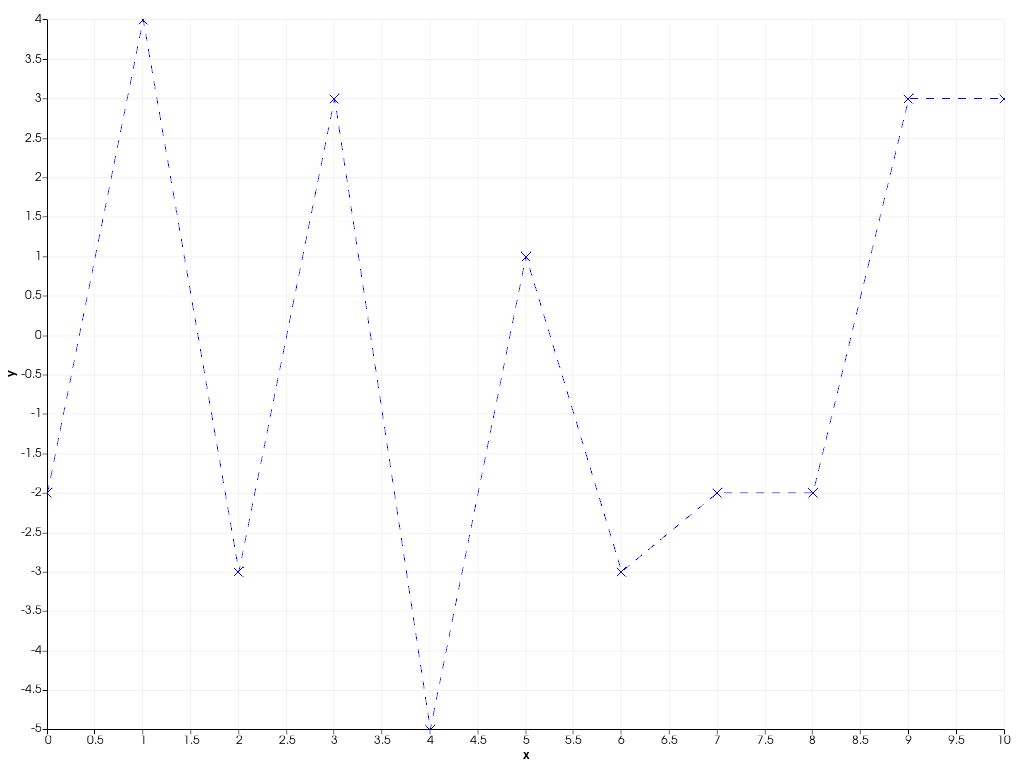The following example shows how to create filled areas between two polylines.

```x = np.linspace(0, 10, 1000)
y1 = np.cos(x) + np.sin(3 * x)
y2 = 0.1 * (x - 5)
chart = pv.Chart2D()
chart.area(x, y1, y2, color=(0.1, 0.1, 0.9, 0.5))
chart.line(x, y1, color=(0.9, 0.1, 0.1), width=4, style="--")
chart.line(x, y2, color=(0.1, 0.9, 0.1), width=4, style="--")
chart.title = "Area plot"  # Set custom chart title
chart.show()
```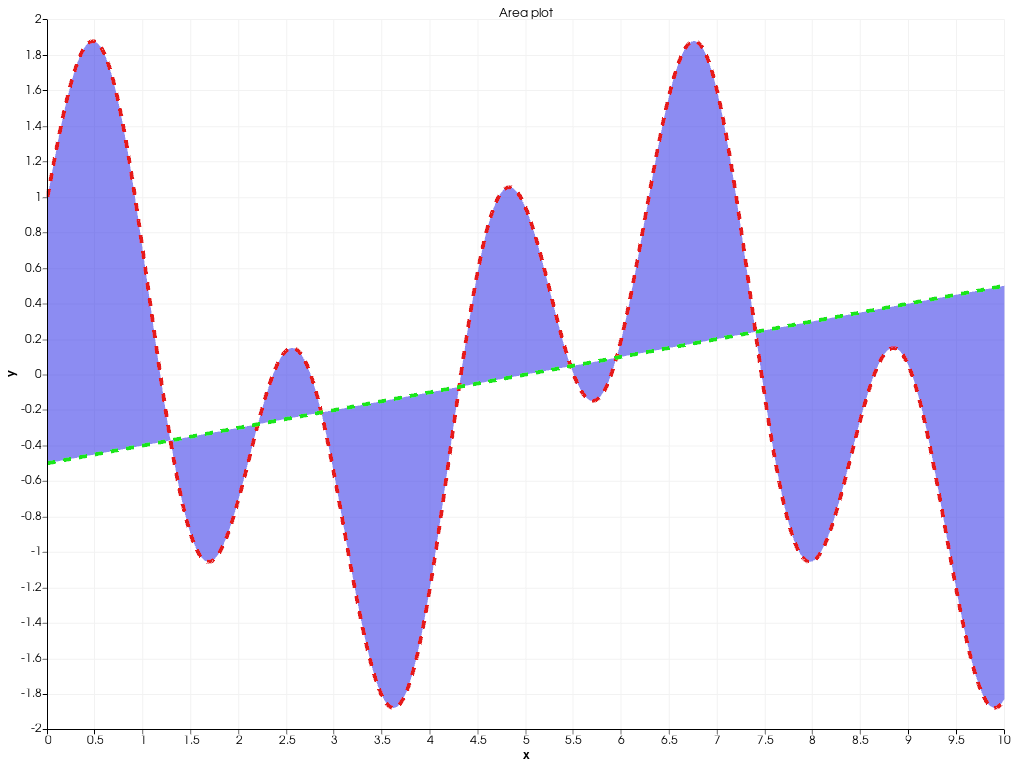Bar charts are also supported. Multiple bar plots are placed next to each other.

```x = np.arange(1, 13)
y1 = rng.integers(1e2, 1e4, 12)
y2 = rng.integers(1e2, 1e4, 12)
chart = pv.Chart2D()
chart.bar(x, y1, color="b", label="2020")
chart.bar(x, y2, color="r", label="2021")
chart.x_axis.tick_locations = x
chart.x_axis.tick_labels = [
"Jan",
"Feb",
"Mar",
"Apr",
"May",
"Jun",
"Jul",
"Aug",
"Sep",
"Oct",
"Nov",
"Dec",
]
chart.x_label = "Month"
chart.y_axis.tick_labels = "2e"
chart.y_label = "# incidents"
chart.show()
```In case you want to stack the bars, instead of drawing them next to each other, pass a sequence of y values.

```x = np.arange(1, 11)
ys = [rng.integers(1, 11, 10) for _ in range(5)]
labels = [f"Machine {i}" for i in range(5)]
chart = pv.Chart2D()
chart.bar(x, ys, label=labels)
chart.x_axis.tick_locations = x
chart.x_label = "Configuration"
chart.y_label = "Production"
chart.grid = False  # Disable the grid lines
chart.show()
```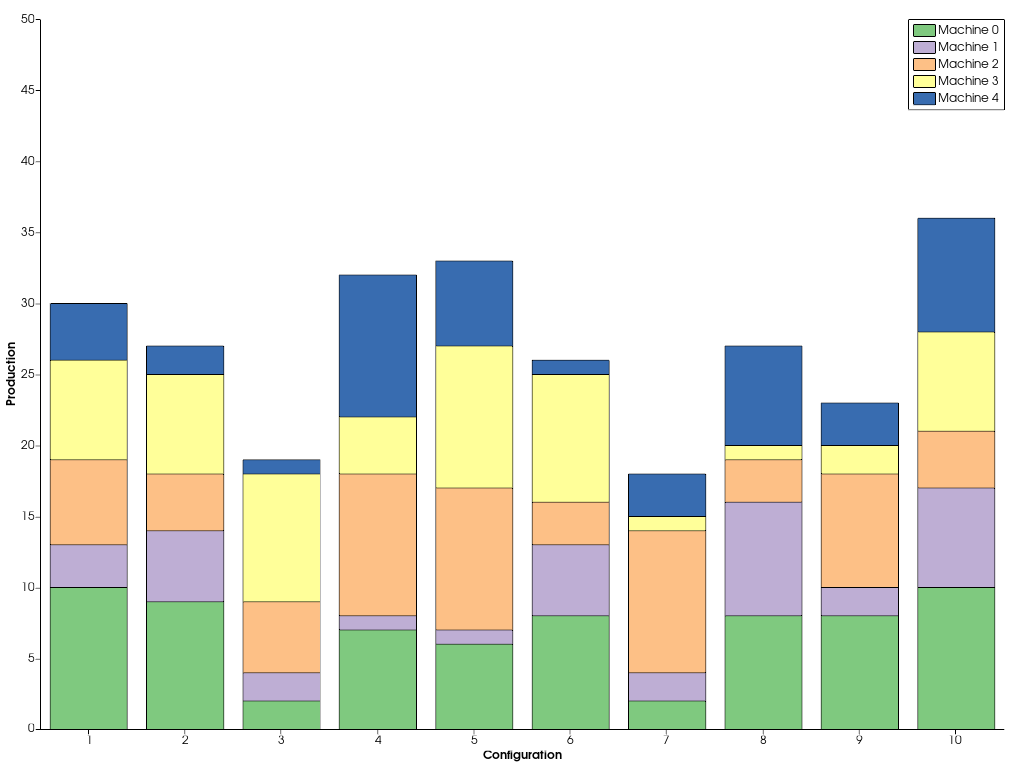In a similar way, you can stack multiple area plots on top of each other.

```x = np.arange(0, 11)
ys = [rng.integers(1, 11, 11) for _ in range(5)]
labels = [f"Segment {i}" for i in range(5)]
chart = pv.Chart2D()
chart.stack(x, ys, labels=labels)
chart.show()
```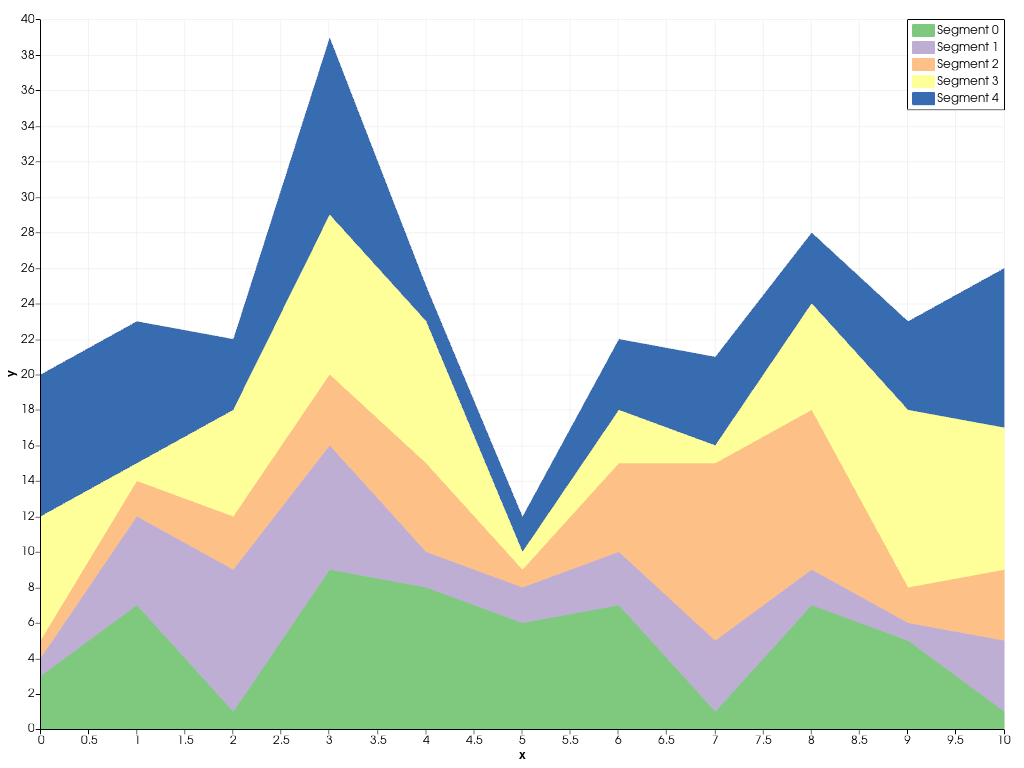Beside the flexible Chart2D used in the previous examples, there are a couple other dedicated charts you can create. The example below shows how a pie chart can be created.

```data = np.array([8.4, 6.1, 2.7, 2.4, 0.9])
chart = pv.ChartPie(data)
chart.plot.labels = [f"slice {i}" for i in range(len(data))]
chart.show()
```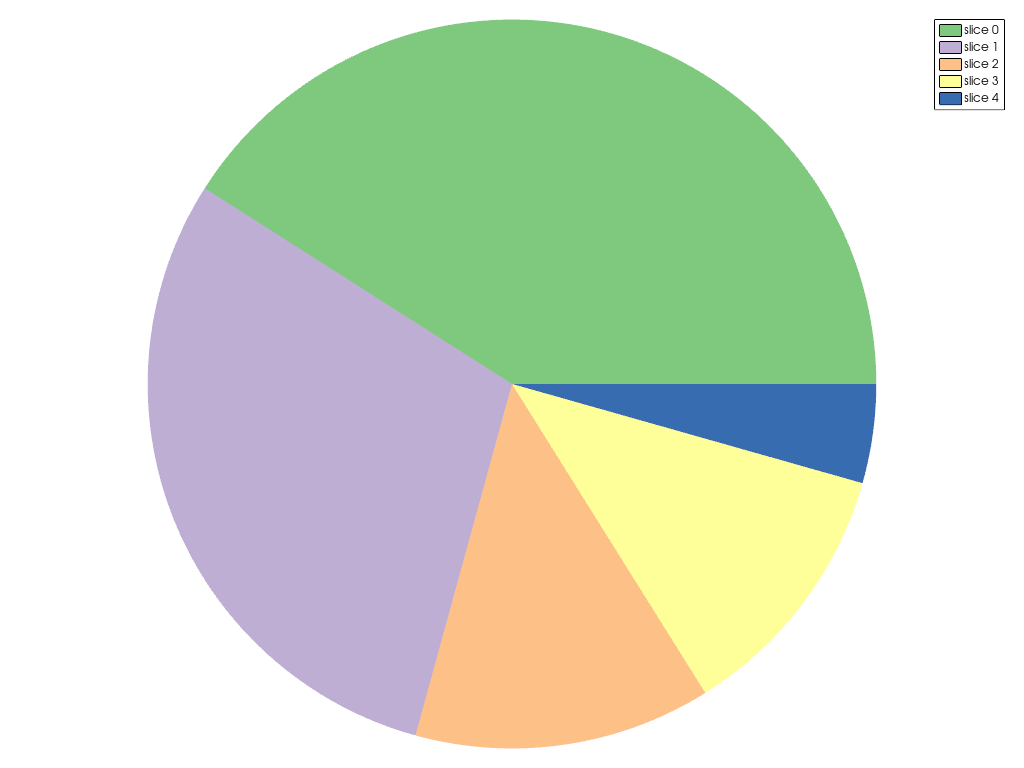To summarize statistics of datasets, you can easily create a boxplot.

```data = [rng.poisson(lam, 20) for lam in range(2, 12, 2)]
chart = pv.ChartBox(data)
chart.plot.labels = [f"Experiment {i}" for i in range(len(data))]
chart.show()
```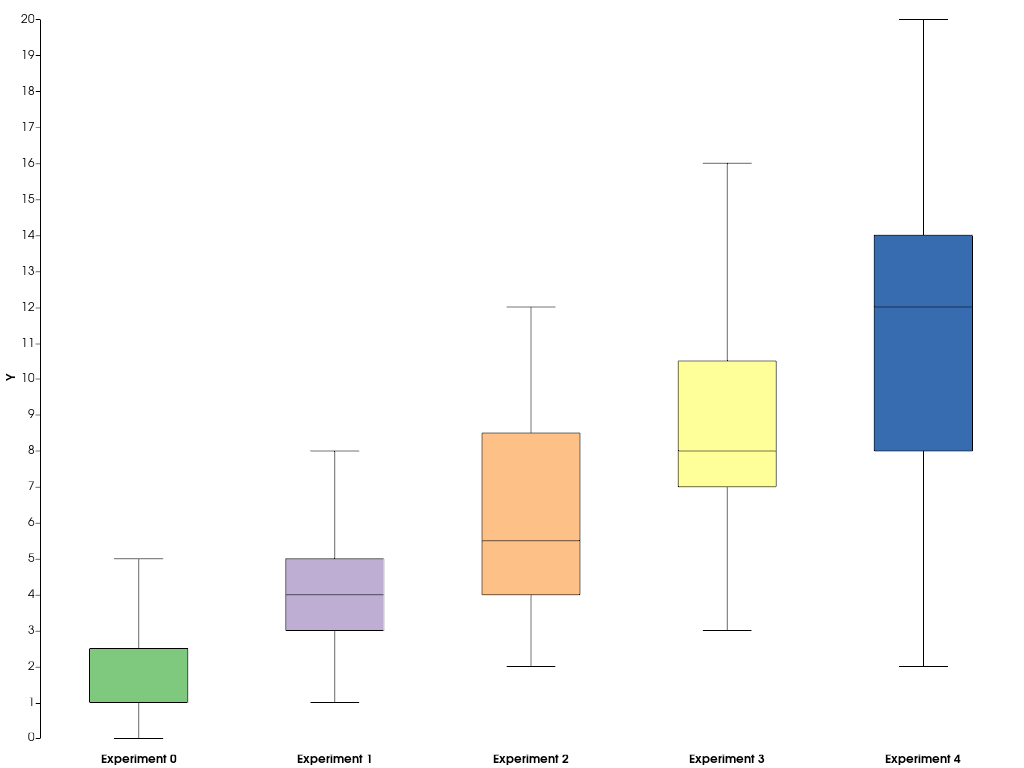If you would like to add other types of chart that are currently not supported by pyvista or VTK, you can resort to matplotlib to create your custom chart and afterwards embed it into a pyvista plotting window. The below example shows how you can do this.

```import matplotlib.pyplot as plt

# First, create the matplotlib figure
f, ax = plt.subplots(
tight_layout=True
)  # Tight layout to keep axis labels visible on smaller figures
alphas = [0.5 + i for i in range(5)]
betas = [*reversed(alphas)]
N = int(1e4)
data = [rng.beta(alpha, beta, N) for alpha, beta in zip(alphas, betas)]
labels = [f"\$\\alpha={alpha:.1f}\\,;\\,\\beta={beta:.1f}\$" for alpha, beta in zip(alphas, betas)]
ax.violinplot(data)
ax.set_xticks(np.arange(1, 1 + len(labels)))
ax.set_xticklabels(labels)
ax.set_title("\$B(\\alpha, \\beta)\$")

# Next, embed the figure into a pyvista plotting window
p = pv.Plotter()
chart = pv.ChartMPL(f)
chart.background_color = 'w'